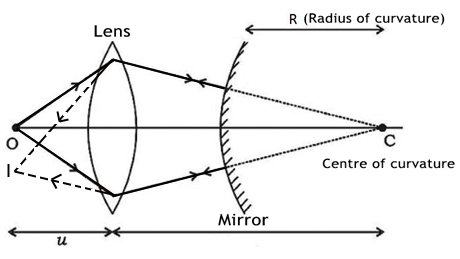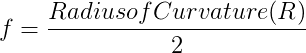Convex Mirror - Focal Length

# Our Objective:

To find the focal length of a convex mirror using a convex lens.

# The Theory:

## Convex mirror

A convex mirror is a curved mirror in which the reflecting surface bulges towards the light source. Convex mirrors reflect light outwards; therefore they are not used to focus light. A convex mirror is also known as fish eye mirror or diverging mirror.
The image formed by a convex lens is virtual and erect, since the focal point (F) and the centre of curvature (2F) are both imaginary points "inside" the mirror that cannot be reached. As a result, images formed by these mirrors cannot be projected on a screen, since the image is inside the mirror. Therefore, its focal length cannot be determined directly. The image is smaller than the object, but gets larger as the object approaches the mirror. The ray diagram of a convex mirror is shown below.The focal length of a convex mirror can be determined by introducing a convex lens between the object and the convex mirror. An image can be obtained with the help of a convex lens side by side with the object when the convex mirror reflects the rays along the same path, i.e., when the rays fall normally on the mirror. Then, the radius of curvature, R, of the mirror is the distance between the screen and the mirror.The focal length f of the convex mirror is calculated using the formula,# Learning Outcomes:

Students understand the following terms:

• Convex mirror
• Focal point